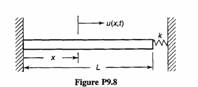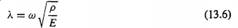### Create an Account

Home / Questions / Consider axial free vibration of the uniform bar AE constant pA constant in Fig P9.8 The...

# Consider axial free vibration of the uniform bar AE constant pA constant in Fig P9.8 The stiffness of the linear spring at x L is k AEf2L ignore its mass

Consider axial free vibration of the uniform bar (AE = constant, pA = constant) in Fig. P9.8. The stiffness of the linear spring at x = L is k = AEf2L; ignore its mass, (a) Determine the boundary condition at end x = L. (b) Obtain the characteristic equation from which eigenvalues can be determined. Your answer should contain the parameters λ and L, where λ is defined by Eq. 13.6. (c) Determine an expression for the fundamental axial frequency of the bar (to, rad/s), (d) Determine an expression for the fundamental axial mode shape, φi(x) and use M a t l a b , or another computer program, to plot the fundamental mode. Scale the mode so that its maximum value is 1.0.May 19 2020 View more View LessSubscribe To Get Solution